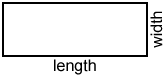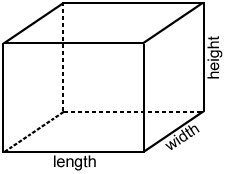# 3D

A three-dimensional space (3D) has three dimensions, such as length, width, and height (or depth). The term "3D" is commonly used to describe shapes and figures in geometry. We live in a 3D world, every object we touch, see, and use are 3D objects. The following is a few examples.

## 3D shapes and figures

While the dimensions of a 2D shape can be described with length and width, a 3D shape requires an additional dimension, often referred to as height or depth.

2D3DThe dimensions of the 2D rectangle above are length and width.The base of the 3D rectangular prism above is a rectangle that gives it two of its dimensions. The height of the prism is perpendicular to its base giving the prism its third dimension.

## 3D Coordinate geometry

Determining the position of a point in 3D is similar to determining the position of a point in 2D, except that there is a third axis, the z-axis, in addition to the x- and y-axes. All three axes are perpendicular to each other, as shown in the figure below.

While the x- and y-axes in 2D are conventionally the horizontal and vertical axes, respectively, in 3D their orientations can vary. As such, when determining which direction to move along any axis, the negative and positive direction must be indicated on the axes, as in the image above. A negative value indicates movement along an axis in the negative direction, and a positive value indicates movement in the positive direction. As such, the point (2, 3, 4) indicates movement in the positive direction along each axis in the coordinate space above.